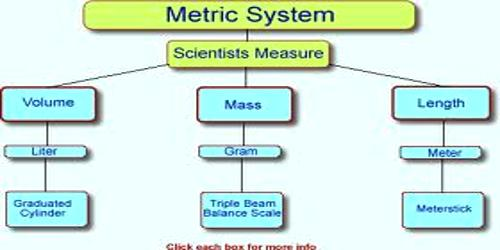Comparing Metric System: Masses, Volumes and Lengths

The metric system uses units such as meter, liter, and gram to measure length, liquid volume, and mass, just as the U.S. customary system uses feet, quarts, and ounces to measure these.

The metric system are used, especially in medical, scientific, and technical fields. In most other countries, the metric system is the primary system of measurement. If you travel to other countries, you will see that road signs list distances in kilometers and milk is sold in liters. People in many countries use words like “kilometer,” “liter,” and “milligram” to measure the length, volume, and weight of different objects. These measurement units are part of the metric system.Comparing Mass in Grams

The metric system has prefix modifiers that are powers of 10.

• A kilogram is 1000 grams
• A hectogram is 100 grams
• A decagram is 10 grams
• A gram is the basic unit of mass
• A decigram is 1/10 gram
• A centigram is 1/100 gram
• A milligram is 1/1000 gram

Comparing Metric Liters

The metric system has prefix modifiers that are powers of 10.

• A kiloliter is 1000 liters
• A hectoliter is 100 liters
• A decaliter is 10 liters
• A liter is the basic unit of volume
• A deciliter is 1/10 liter
• A centiliter is 1/100 liter
• A milliliter is 1/1000 liter

Comparing Metric Length

The metric system has prefix modifiers that are powers of 10.

• A kilometer is 1000 meters
• A hectometer is 100 meters
• A decameter is 10 meters
• A meter is the basic unit of length
• A decimeter is 1/10 meter
• A centimeter is 1/100 meter
• A millimeter is 1/1000 meter

Information Source;